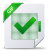# Relaxed algorithms for multiplication

Relaxed algorithms for multiplication

Basic operations Algorithmically, we represent p-adics through their base-M expansion, that is, through a sequence of coefficients in M. Roughly speaking, we measure the cost of an algorithm by the number of arithmetic operations with operands in M it performs. More precisely, we assume that we can do the following at unit cost: • given a0, b0 in M, compute the coefficients c0, c1 of c= a0 b0 at unit cost, and similarly for the coefficients of a ± b • given a0 in M − {0}, compute b0 in M − {0} such that a0 b0 = 1 mod p Remark that when R = k[X], we are simply counting arithmetic operations in k. The main operations we will need on p-adics are sum and difference, as well as multiplication and a few of its variants (of course, these algorithms only operate on truncated p-adics). Addition (and subtraction) are easy to deal with: Lemma 1.1. The following holds: • Given two p-adics a, b of length at most ℓ, one can compute a+b in time O(ℓ) • Given p-adics a1, , aN of length at most ℓ, the p-adic A= P i=1 N ai has length O(log (N) + ℓ), and one can compute it in time O(N ℓ ). • Given p-adics a1, , aN of length at most ℓ, the p-adic A = P i=1 N ai p i has length O(N + ℓ), and one can compute it in time O(N ℓ).

Lire sur cLicours.com :  Cours algorithmique et fondement mathématique

Proof

The first point is easily dealt with by induction on ℓ; we will see the algorithm in more detail in Example 1.5 below. To handle the second one, we build a tree
adder, which has depth O(log (N)). The length bound follows; the complexity bound comes from noticing that we do O(N) additions of p-adics of length ℓ, O(N/2)
additions of p-adics of length ℓ+ 1, O(N/4) additions of p adics of length ℓ+ 2, etc. To deal with the last point, note that for all i, ai p i has length at most ℓ +

1. Using the second point, we deduce the length bound, and the upper bound

O(N (ℓ + N)) on the time it takes to compute the sum. If N 6 ℓ, we are done.
Else, we rewrite the sum as P
j=0
ℓ−1
bj p
j
, where bj is the p-adic of length N whose coefficients are the coefficients of index j of a1, , aN. Thus, we have reversed the roles of ℓ and N, so the claim is valid in all cases.

For multiplication, we will distinguish several variants; for the moment, we will simply define the problems, and introduce notation for their complexity.First, we consider “plain” multiplication: given a and b of length at most n, compute their product (which has length at most 2n). For this operation, we will let I: N→N be such that all coefficients of a b can be computed in I(n) operations.We will assume that I(n) satisfies the property that I(n)/n is non-decreasing. Using Fast Fourier Transform, it is possible to take I(n) quasi-linear , that is, linear up tologarithmic factors: we will review this in the next section.Two related problems will be of interest: short and middle products. The shortproduct at precision n is essentially the product of p-adics modulo pn; precisely, oninput a and b with max (λ(a), λ(b)) = n, it computes the coefficients of SP(a, b) 7 X 06i+j<n ai bj p i+j

The definition of the middle product is slightly more complex: if a and b are p-adics with λ(b) = n, the middle product of a and b is defined as (essentially) the middle part of the product c 7 a b; precisely, it computes

MP(a, b) 7 X

n−16i+j62n−2

ai bj p i+j

.In general, attention must be paid to carries: because of them, MP(a, b) may not consist in exactly the middle coefficients of a b. In the case where R =k[X], though,middle and short products simply compute a few of the coefficients of the product a b, so they can be computed by means of “plain” multiplication algorithms. We will see below that savings are possible: Section 1.2 gives algorithms for short and middle products, with a focus on the important particular case where R = k[X].Télécharger le document complet